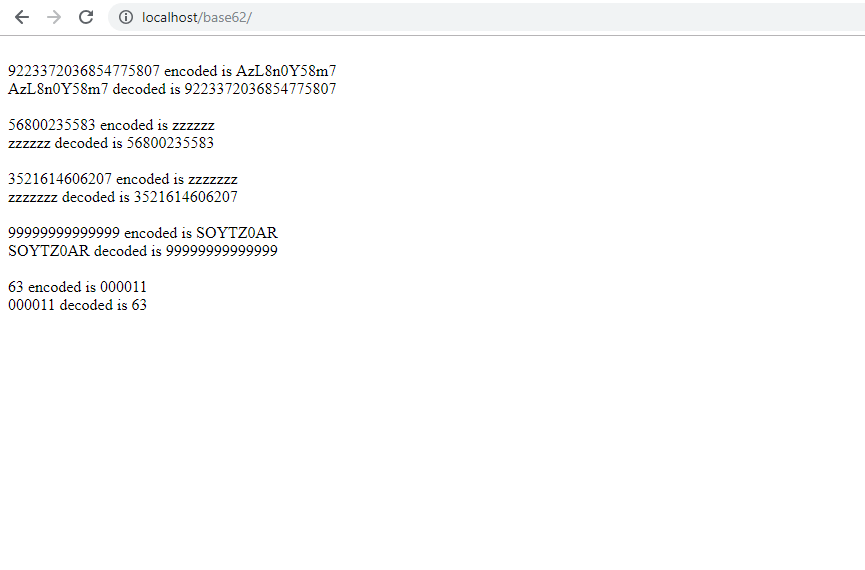# Convert Very Large Decimal Integers to Base 62 And Back

The other day I was trying to devise a way of converting arbitrarily large base 10 integers to the order of a billion billion to base 62 and did not get much help from the web. So I devised my own solution using the BC Math extension which ships with PHP by default. So, this solution works upto PHP_INT_MAX which is 9223372036854775807 for 64-bit platform. It also works upto 99 trillion on 32-bit-platform. I used an array as a stack to store successive remainders of division by the base, which is 62, and at the end popped them one by one from the stack to generate the converted base 62 number. The converse, multiplication by successive powers of 62 and addition of those powers helped to convert base 62 back to base 10.

First I used PHP OOPS to create a class called MyBase62Converter. The code is given below:

```class MyBase62Converter
{
private \$base62StrVar = '0123456789ABCDEFGHIJKLMNOPQRSTUVWXYZabcdefghijklmnopqrstuvwxyz';
private \$baseInt = 62;

function encode(\$decNum)
{
\$base = \$this->baseInt;

\$myStack = array();

\$remainder = 0;

\$base62Str = \$this->base62StrVar;

do
{

\$remainder = bcmod(\$decNum, \$base);

array_push(\$myStack, \$remainder);

\$decNum = bcdiv(bcsub(\$decNum, \$remainder), \$base);

}
while(\$decNum > 0);

\$binNum = '';

while(count(\$myStack) > 0)
{

\$binNum.= substr(\$base62Str, array_pop(\$myStack), 1);
}

}

function decode(\$baseNum)
{

\$base62Str = \$this->base62StrVar;

\$base = \$this->baseInt;

\$hashLen = strlen(\$baseNum);

\$decNum = '';

for(\$i = 0; \$i < \$hashLen; \$i++)
{
\$decNum = bcadd(\$decNum, bcmul(bcpow(\$base, \$i), strpos(\$base62Str , substr(\$baseNum, \$hashLen - \$i - 1, 1))));
}

return \$decNum;
}

}

```

Using the two simple encode and decode functions we can convert base 10 integers to base 62 representation and vice versa.

You can see the example below:

```require_once("lib/class.MyBase62Converter.php");

\$encoder = new MyBase62Converter();

echo "<br />";

echo PHP_INT_MAX." encoded is ".\$encoded = \$encoder->encode(PHP_INT_MAX);

echo "<br />";

echo \$encoded." decoded is ".\$decoded = \$encoder->decode(\$encoded);

echo "<br />";

\$largeNum = bcpow(62, 6) - 1;

echo \$largeNum." encoded is ".\$encoded = \$encoder->encode(\$largeNum);

echo "<br />";

echo \$encoded." decoded is ".\$decoded = \$encoder->decode(\$encoded);

echo "<br />";

\$veryLargeNum = bcpow(62, 7) - 1; //getting 0 due to num expressed in scientfic exponential notation 2.183401055849E+14

echo \$veryLargeNum." encoded is ".\$encoded = \$encoder->encode(\$veryLargeNum);

echo "<br />";

echo \$encoded." decoded is ".\$decoded = \$encoder->decode(\$encoded);

echo "<br  />";

\$max_num = 99999999999999;

echo \$max_num." encoded is ".\$encoded = \$encoder->encode(\$max_num , 62); //99999999999999

echo "<br />";

echo \$encoded." decoded is ".\$decoded = \$encoder->decode(\$encoded);

echo "<br />";

\$new_num = 63;

echo \$new_num." encoded is ".\$encoded = \$encoder->encode(\$new_num , 62);

echo "<br />";

echo \$encoded." decoded is ".\$decoded = \$encoder->decode(\$encoded);
```

The result of code execution is given below:## 3 thoughts on “Convert Very Large Decimal Integers to Base 62 And Back”

1.Jamestrine says:

Hi,

I’ve been visiting your website a few times and decided to give you some positive feedback because I find it very useful. Well done.

I was wondering if you as someone with experience of creating a useful website could help me out with my new site by giving some feedback about what I could improve?

You can find my site by searching for “casino gorilla” in Google (it’s the gorilla themed online casino comparison).

I would appreciate if you could check it out quickly and tell me what you think.

casinogorilla.com

Thank you for help and I wish you a great week!